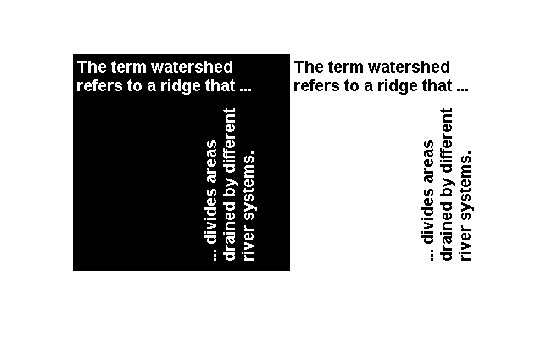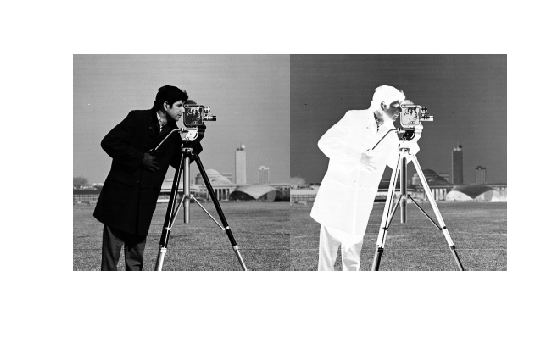# imcomplement

Complement image

## Syntax

``J = imcomplement(I)``

## Description

example

````J = imcomplement(I)` computes the complement of the image `I` and returns the result in `J`.```

## Examples

collapse all

```X = uint8([ 255 10 75; 44 225 100]); X2 = imcomplement(X)```
```X2 = 2x3 uint8 matrix 0 245 180 211 30 155 ```
```bw = imread('text.png'); bw2 = imcomplement(bw); imshowpair(bw,bw2,'montage')``````I = imread('cameraman.tif'); J = imcomplement(I); imshowpair(I,J,'montage')```Read a color image into the workspace.

```rgb = imread('yellowlily.jpg'); imshow(rgb)```Display the complement of the image.

```c = imcomplement(rgb); imshow(c)```Each color channel of the resulting image is the complement of the corresponding color channel in the original image. Regions that were dark, such as dirt, become light. In the original image, the leaves appear green, and petals appear yellow because of a mixture of red and green signals. In the complement image, the leaves appear purple because the red and blue signals are larger than the green signal. The flower petals appear blue because the blue signal is larger than the red and green channels.

## Input Arguments

collapse all

Input image, specified as an RGB, grayscale, or binary image.

Data Types: `single` | `double` | `int8` | `int16` | `int32` | `uint8` | `uint16` | `uint32` | `logical`

## Output Arguments

collapse all

Image complement, specified as an RGB, grayscale, or binary image. `J` has the same size and class as the input image, `I`.

collapse all

### Image Complement

In the complement of a binary image, zeros become ones and ones become zeros. Black and white are reversed.

In the complement of a grayscale or color image, each pixel value is subtracted from the maximum pixel value supported by the class (or 1.0 for double-precision images). The difference is used as the pixel value in the output image. In the output image, dark areas become lighter and light areas become darker. For color images, reds become cyan, greens become magenta, blues become yellow, and vice versa.

## Tips

• If `I` is a grayscale or RGB image of class `double`, then you can use the expression `1-I` instead of this function.

• If `I` is a binary image, then you can use the expression `~I` instead of this function.

## Version History

Introduced before R2006a